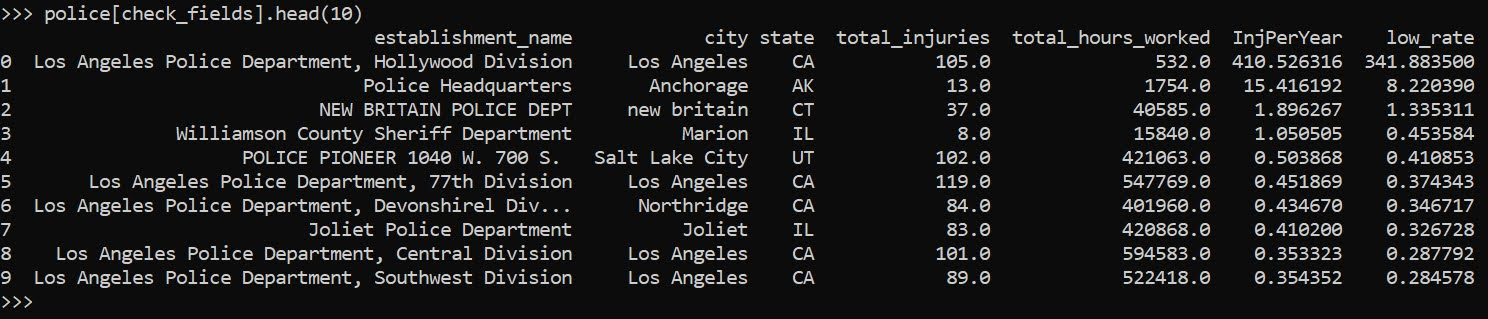# Injury rates at Amazon warehouses

I follow several of the News & Observer (The Raleigh/Durham newspaper) newsletters, and Brian Gordon and Tyler Dukes had a story recently about fainting and ambulance runs at the Amazon warehouse in Raleigh, Open Source: Ambulances at Amazon. He did some great sleuthing, and showed that while the number on its face seemed high (an ambulance call around 1 out of 3 days) the rate of ambulance runs when accounting for the size of the workforce is pretty similar to other warehouses.

Here I will show an example of downloading the OSHA injury data to show a similar finding. Using python it is pretty quick work.

So first can import the libraries we need (the typical scientific stack). I download the OSHA data for 2021, and I calculate injury rates per person work year, so how to interpret these are at the workplace level. Per full time people per year, it is the expected number of injuries across the workforce.

``````import pandas as pd
import matplotlib.pyplot as plt
import numpy as np
from scipy.stats import beta

inj_2021url = "https://www.osha.gov/sites/default/largefiles/ITA-data-cy2021.zip"
# Calculate injuries per person full year
inj_dat['InjPerYear'] = (inj_dat['total_injuries']/inj_dat['total_hours_worked'])*2080``````

We can filter out warehouse workers via NAICS code `493110`. I also just limit to warehouses in North Carolina. Sorting by the injury rate, Amazon is not even in the top 10 in the state:

``````warehouses = inj_dat[inj_dat['naics_code'] == 493110].copy()
warehouses_nc = warehouses[warehouses['state'] == 'NC'].reset_index(drop=True)
warehouses_nc['AmazonFlag'] = 1*(warehouses_nc['company_name'].str.find('Amazon.com') >= 0)

# Rate per year of work per person, 2080
warehouses_nc.sort_values('InjPerYear',ascending=False,ignore_index=True,inplace=True)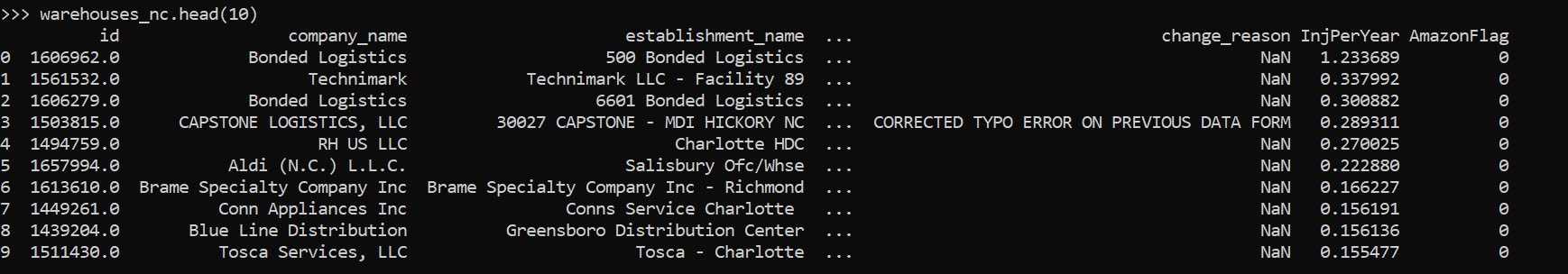But note that I don’t think Bonded Logistics is a terribly dangerous place. One thing you need to watch out for when evaluating rate data is that places with smaller denominators (here lower total hours worked) tend to be more volatile. So a useful plot is to plot the total hours work (cumulative for the entire warehouse) against the overall rate of injuries per hour worked.

``````fig, ax = plt.subplots(figsize=(12,6))
ax.scatter(nam_ware['total_hours_worked'], nam_ware['InjPerYear'],
c='grey', s=30, edgecolor='k', alpha=0.5, label='Other Warehouses')
ax.scatter(amz_ware['total_hours_worked'], amz_ware['InjPerYear'],
c='blue', s=80, edgecolor='k', alpha=0.9, label='Amazon Warehouses')
ax.set_axisbelow(True)
ax.set_xlabel('Total Warehouse Hours Worked')
ax.set_ylabel('Injury Rate per Person Work Year (2080 hours)')
ax.legend(loc='upper right')
plt.savefig('InjRate.png', dpi=500, bbox_inches='tight')``````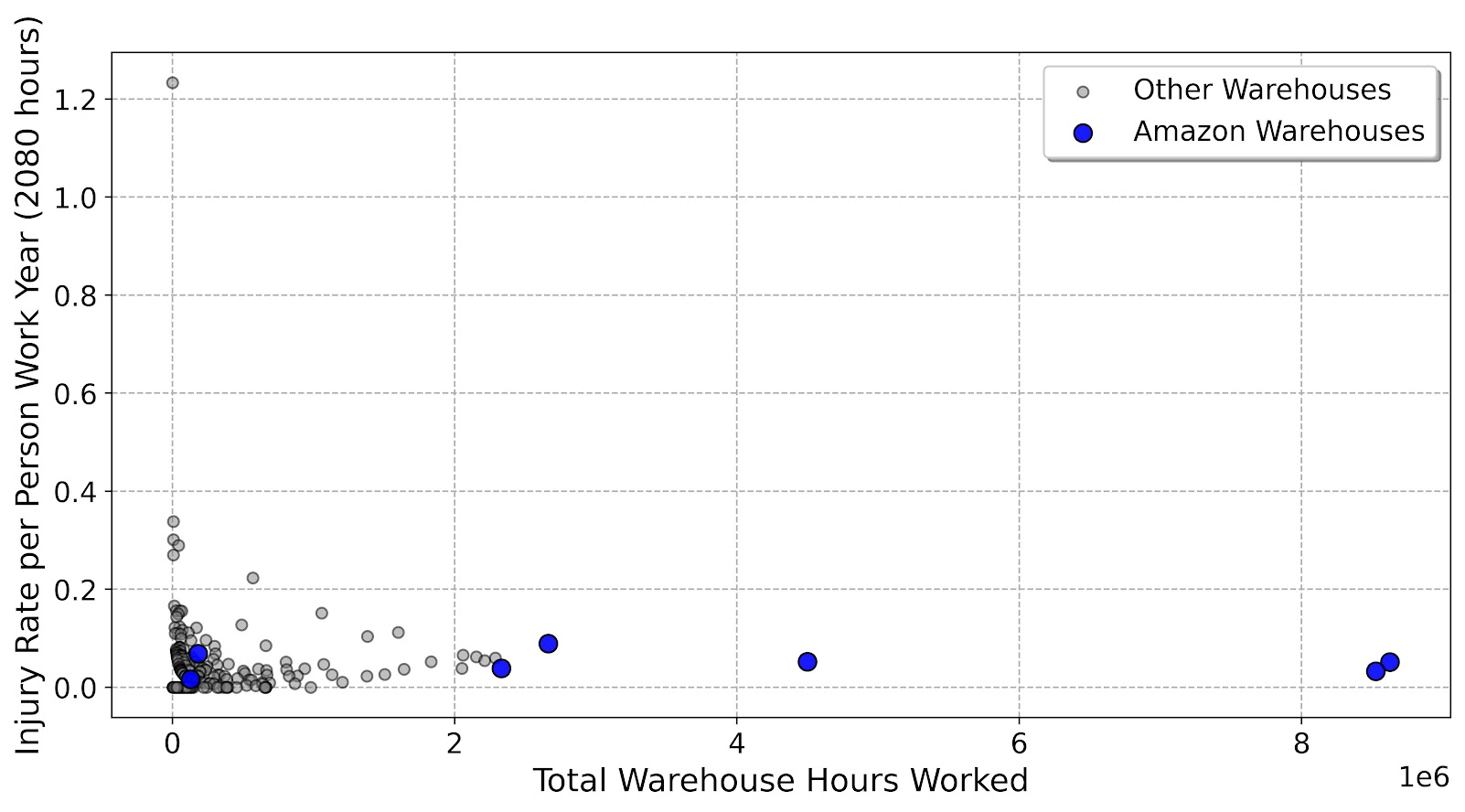You can see by this plot the Amazon warehouses have the largest total number of hours worked (by quite a few) relative to many other warehouses in North Carolina. But their overall rate of injuries is right in line with the rest of the crowd. Looking at the overall rate, it is around `0.04` (so you would expect around 1/20 full time workers to have an injury per year at a warehouse according to this data).

``````tot_rate = warehouses_nc['total_injuries'].sum()/warehouses_nc['total_hours_worked'].sum()
print(tot_rate*2080)``````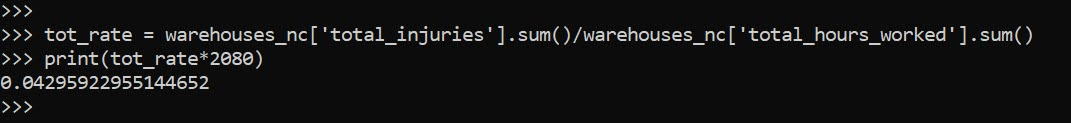If we do this plot again, but add funnel bound lines to show the typical volatility we would expect with estimating these rates:

``````# Binomial confidence interval
def binom_int(num,den,confint=0.95):
quant = (1 - confint)/ 2.
low = beta.ppf(quant, num, den - num + 1)
high = beta.ppf(1 - quant, num + 1, den - num)
return (np.nan_to_num(low), np.where(np.isnan(high), 1, high))

den = np.geomspace(1000,8700000,500)
num = den*tot_rate
low_int, high_int = binom_int(num,den,0.99)
high_int = high_int*2080

fig, ax = plt.subplots(figsize=(12,6))
ax.plot(den,high_int, c='k', linewidth=0.5)
ax.hlines(tot_rate*2080,1000,8700000,colors='k', linewidths=0.5)
ax.scatter(nam_ware['total_hours_worked'], nam_ware['InjPerYear'],
c='grey', s=30, edgecolor='k', alpha=0.5, label='Other Warehouses')
ax.scatter(amz_ware['total_hours_worked'], amz_ware['InjPerYear'],
c='blue', s=80, edgecolor='k', alpha=0.5, label='Amazon Warehouses')
ax.set_axisbelow(True)
ax.set_xlabel('Total Warehouse Hours Worked')
ax.set_ylabel('Injury Rate per Person Work Year (2080 hours)')
plt.xscale('log', basex=10)
ax.legend(loc='upper right')
ax.annotate('Straight line is average overall injury rate\nCurved line is Binomial 99% Interval',
xy = (0.00, -0.13), xycoords='axes fraction')
plt.savefig('InjRate_wBin.png', dpi=500, bbox_inches='tight')``````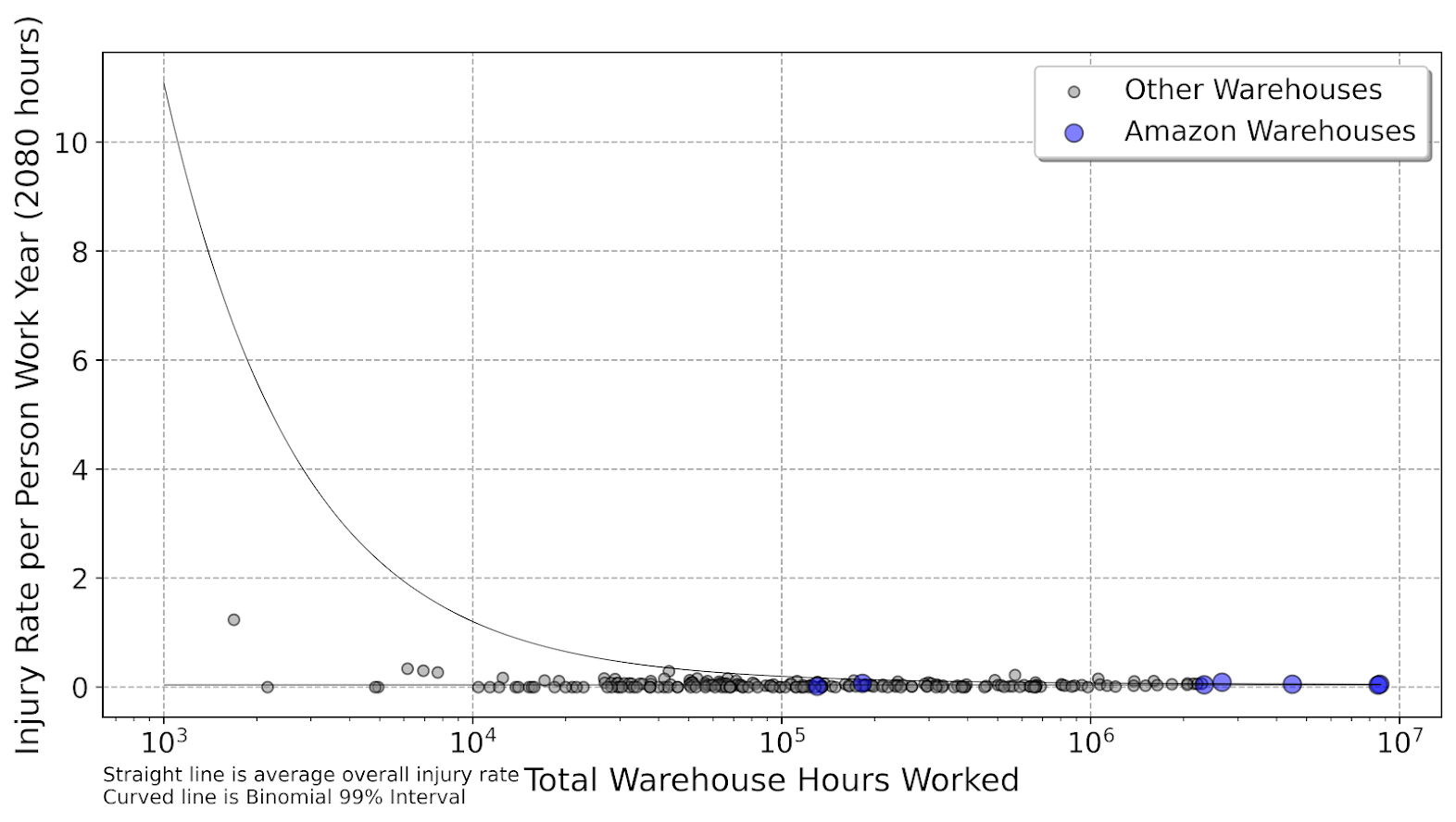So you can see even Bonded Logistics is well within the average rate you would expect to still be consistent with the average overall injury rate relative to all the other warehouses in North Carolina.

As a note, I imagine I saw someone using this data recently looking at police departments in a criminal justice paper (I have in my notes police departments are NAICS code `922120`). (Maybe Justin Nix/Michael Sierra-Arévalo/Ian Adams?) But sorry do not remember the paper (so I owe credit to someone else for pointing out this data, but not sure who).

Another way to do the analysis is to calculate the lower/upper confidence intervals per the rates, and then sort by the lower confidence interval. This way you can filter out high rate variance locations.

``````# Can look at police departments
# NAICS code 922120
police = inj_dat[inj_dat['naics_code'] == 922120].copy()
low_police, high_police = binom_int(police['total_injuries'],police['total_hours_worked'])
police['low_rate'] = low_police*2080
police.sort_values('low_rate',ascending=False,ignore_index=True,inplace=True)
check_fields = ['establishment_name','city','state','total_injuries','total_hours_worked','InjPerYear','low_rate']